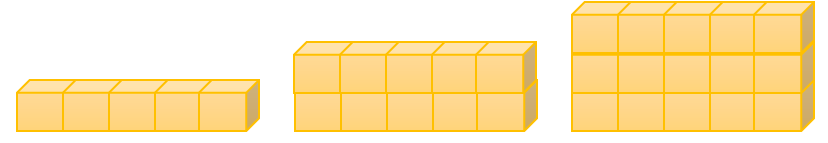General
General
Easy

Question

# Jin creates a pattern using the rule “Each layer has 5-blocks”.The number of cubes in the next shape in Jin’s pattern is

## 18    20    24    25Hint:

## The correct answer is: 20

### We are given a pattern with number of cubes. The rule used to make the pattern is “Each layer has five blocks”.One layer is added every time. With each layer, 5 blocks are added.The number of cubes in the first shape is 5.The number of cubes in the second shape is (2 × 5) = 10The number of cubes in the third shape is (3 × 5) = 15So, the number of cubes in the fourth shape will be (4 × 5 ) = 20The number of cubes in next shape are 20.

We have to be careful about the order of the shapes.

### Related Questions to study#### With Turito Foundation.#### Get an Expert Advice From Turito.